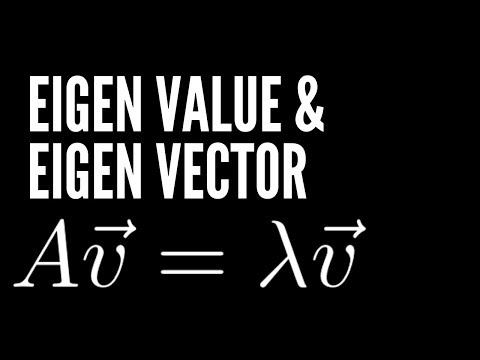# Visualizing Eigenvectors & Eigenvalues using Python

Eigenvalue & eigenvector are probably one of the most important concepts in linear algebra. Who can expect a simple equation like Av = λv is so significant? From machine learning, quantum computing, and physic, many mathematical and engineering problems can be solved by finding the eigenvalue and eigenvectors of a matrix.

Let’s not only discover what it is but also visualize it using Python.

## To view the video